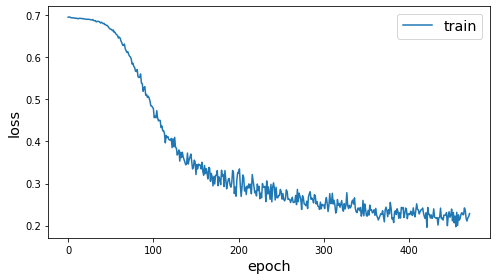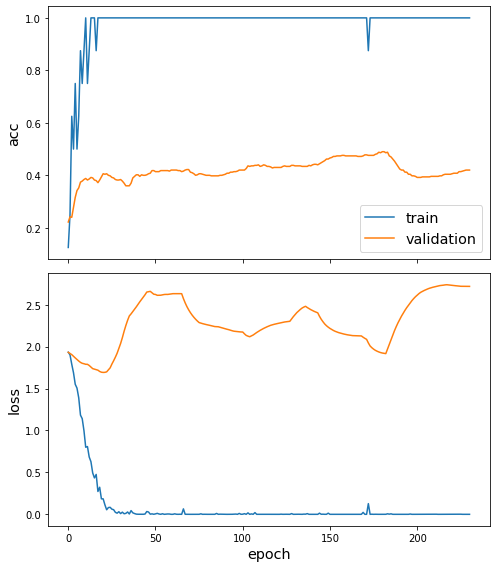# Semi-supervised node classification via GCN, Deep Graph Infomax and fine-tuning¶

This demo demonstrates how to perform semi-supervised node classification, using the Deep Graph Infomax algorithm and GCN on the Cora dataset. It uses very few labelled training examples, demonstrating the benefits of pre-training a model with Deep Graph Infomax for data scarce environments.

Other related demos:

This follows the usual StellarGraph workflow:

2. create our data generators

3. train our model

We do step 3 three times:

1. Pre-train a GCN model using Deep Graph Infomax, without any labelled data

2. Fine-tune that GCN model using the small training set

3. Train a fresh GCN model from scratch with the training set (no pre-training).

:

import stellargraph as sg
from stellargraph.mapper import CorruptedGenerator, FullBatchNodeGenerator
from stellargraph.layer import GCN, DeepGraphInfomax

import pandas as pd
from sklearn import model_selection, preprocessing
from IPython.display import display, HTML

import tensorflow as tf
from tensorflow.keras import Model, layers, optimizers, callbacks


:

dataset = sg.datasets.Cora()
display(HTML(dataset.description))

The Cora dataset consists of 2708 scientific publications classified into one of seven classes. The citation network consists of 5429 links. Each publication in the dataset is described by a 0/1-valued word vector indicating the absence/presence of the corresponding word from the dictionary. The dictionary consists of 1433 unique words.
:

print(G.info())

StellarGraph: Undirected multigraph
Nodes: 2708, Edges: 5429

Node types:
paper: 
Features: float32 vector, length 1433
Edge types: paper-cites->paper

Edge types:
paper-cites->paper: 
Weights: all 1 (default)


## Data Generators¶

Now we create the data generators using CorruptedGenerator (docs). CorruptedGenerator returns shuffled node features along with the regular node features and we train our model to discriminate between the two.

Note that:

• We typically pass all nodes to corrupted_generator.flow because this is an unsupervised task

• We don’t pass targets to corrupted_generator.flow because these are binary labels (true nodes, false nodes) that are created by CorruptedGenerator

:

fullbatch_generator = FullBatchNodeGenerator(G)

corrupted_generator = CorruptedGenerator(fullbatch_generator)
gen = corrupted_generator.flow(G.nodes())

Using GCN (local pooling) filters...


## Model pre-training with Deep Graph Infomax¶

We create and train our GCN (docs) and DeepGraphInfomax (docs) models. Note that the loss used here must always be tf.nn.sigmoid_cross_entropy_with_logits.

:

def make_gcn_model():
# function because we want to create a second one with the same parameters later
return GCN(
layer_sizes=[16, 16],
activations=["relu", "relu"],
generator=fullbatch_generator,
dropout=0.4,
)

pretrained_gcn_model = make_gcn_model()

:

infomax = DeepGraphInfomax(pretrained_gcn_model, corrupted_generator)
x_in, x_out = infomax.in_out_tensors()

dgi_model = Model(inputs=x_in, outputs=x_out)
dgi_model.compile(
)

:

epochs = 500

:

dgi_es = callbacks.EarlyStopping(monitor="loss", patience=50, restore_best_weights=True)
dgi_history = dgi_model.fit(gen, epochs=epochs, verbose=0, callbacks=[dgi_es])

:

sg.utils.plot_history(dgi_history)## Node classification¶

We’ve now initialised the weights of the model to capture useful properties of the graph structure and node structure. We can now further train the model to perform a node classification prediction task. To emphasise the value of the unsupervised weights, we will use a very small amount of labelled data for training.

See the GCN node classification demo for more details on this task.

### Data preparation¶

The Cora dataset labels academic papers into one of 7 subjects:

:

node_classes.value_counts().to_frame()

:

subject
Neural_Networks 818
Probabilistic_Methods 426
Genetic_Algorithms 418
Theory 351
Case_Based 298
Reinforcement_Learning 217
Rule_Learning 180

To simulate a data-poor environment, we will split the data into a train set of size 8, along with test and validation sets.

:

train_classes, test_classes = model_selection.train_test_split(
node_classes, train_size=8, stratify=node_classes, random_state=1
)
val_classes, test_classes = model_selection.train_test_split(
test_classes, train_size=500, stratify=test_classes
)


The train set has only one or two observations of each class.

:

train_classes.value_counts().to_frame()

:

subject
Neural_Networks 2
Probabilistic_Methods 1
Rule_Learning 1
Reinforcement_Learning 1
Genetic_Algorithms 1
Theory 1
Case_Based 1

For a categorical task, the categories need to be one hot encoded.

:

target_encoding = preprocessing.LabelBinarizer()

train_targets = target_encoding.fit_transform(train_classes)
val_targets = target_encoding.transform(val_classes)
test_targets = target_encoding.transform(test_classes)

:

train_gen = fullbatch_generator.flow(train_classes.index, train_targets)
test_gen = fullbatch_generator.flow(test_classes.index, test_targets)
val_gen = fullbatch_generator.flow(val_classes.index, val_targets)


### Fine-tuning model¶

We now have the required pieces to finalise our GCN model for node classification:

• a GCN model with weights pre-trained with Deep Graph Infomax to capture the graph structure

• a small train set

We use the same GCN model as before but train it for a supervised categorical prediction task. See the fully-supervised GCN node classification demo for more details.

:

pretrained_x_in, pretrained_x_out = pretrained_gcn_model.in_out_tensors()

pretrained_predictions = tf.keras.layers.Dense(
units=train_targets.shape, activation="softmax"
)(pretrained_x_out)

:

pretrained_model = Model(inputs=pretrained_x_in, outputs=pretrained_predictions)
pretrained_model.compile(
)

:

prediction_es = callbacks.EarlyStopping(
monitor="val_acc", patience=50, restore_best_weights=True
)

:

pretrained_history = pretrained_model.fit(
train_gen,
epochs=epochs,
verbose=0,
validation_data=val_gen,
callbacks=[prediction_es],
)

:

sg.utils.plot_history(pretrained_history)We’ve now fine-tuned our model for node classification. Observe that the accuracy in the first few epochs was very poor, but it quickly improved. (The train accuracy plot is quantised because the training set is so small.)

:

pretrained_test_metrics = dict(
zip(pretrained_model.metrics_names, pretrained_model.evaluate(test_gen))
)
print(pretrained_test_metrics)

1/1 [==============================] - 0s 920us/step - loss: 1.5896 - acc: 0.5632
{'loss': 1.5896106958389282, 'acc': 0.5631818175315857}


## Model without Deep Graph Infomax pre-training¶

Let’s also train an equivalent GCN model in a fully supervised manner, starting with the same model configuration and using the same 8 training examples.

:

direct_gcn_model = make_gcn_model()
direct_x_in, direct_x_out = direct_gcn_model.in_out_tensors()
direct_predictions = tf.keras.layers.Dense(
units=train_targets.shape, activation="softmax"
)(direct_x_out)

:

direct_model = Model(inputs=direct_x_in, outputs=direct_predictions)
direct_model.compile(
)

:

direct_history = direct_model.fit(
train_gen,
epochs=epochs,
verbose=0,
validation_data=val_gen,
callbacks=[prediction_es],
)

:

sg.utils.plot_history(direct_history):

direct_test_metrics = dict(
zip(direct_model.metrics_names, direct_model.evaluate(test_gen))
)
print(direct_test_metrics)

1/1 [==============================] - 0s 946us/step - loss: 2.0196 - acc: 0.4559
{'loss': 2.0196211338043213, 'acc': 0.4559091031551361}


## Comparison of model performance¶

The following table shows the performance of the two models, for comparison.

:

pd.DataFrame(
[pretrained_test_metrics, direct_test_metrics],
index=["with DGI pre-training", "without pre-training"],
).round(3)

:

loss acc
with DGI pre-training 1.59 0.563
without pre-training 2.02 0.456

## Conclusion¶

In this demo, we performed semi-supervised node classification on the Cora dataset. This example had extreme data scarcity: only 8 labelled training examples, with one or two from each of the 7 classes. We used Deep Graph Infomax to train a GCN model on the whole Cora graph, without labels. We then further trained this GCN model in the normal manner, to fine-tuned its weights on the small set of labelled data. The GCN model pre-trained with Deep Graph Infomax outperforms a GCN model without any such pre-training.